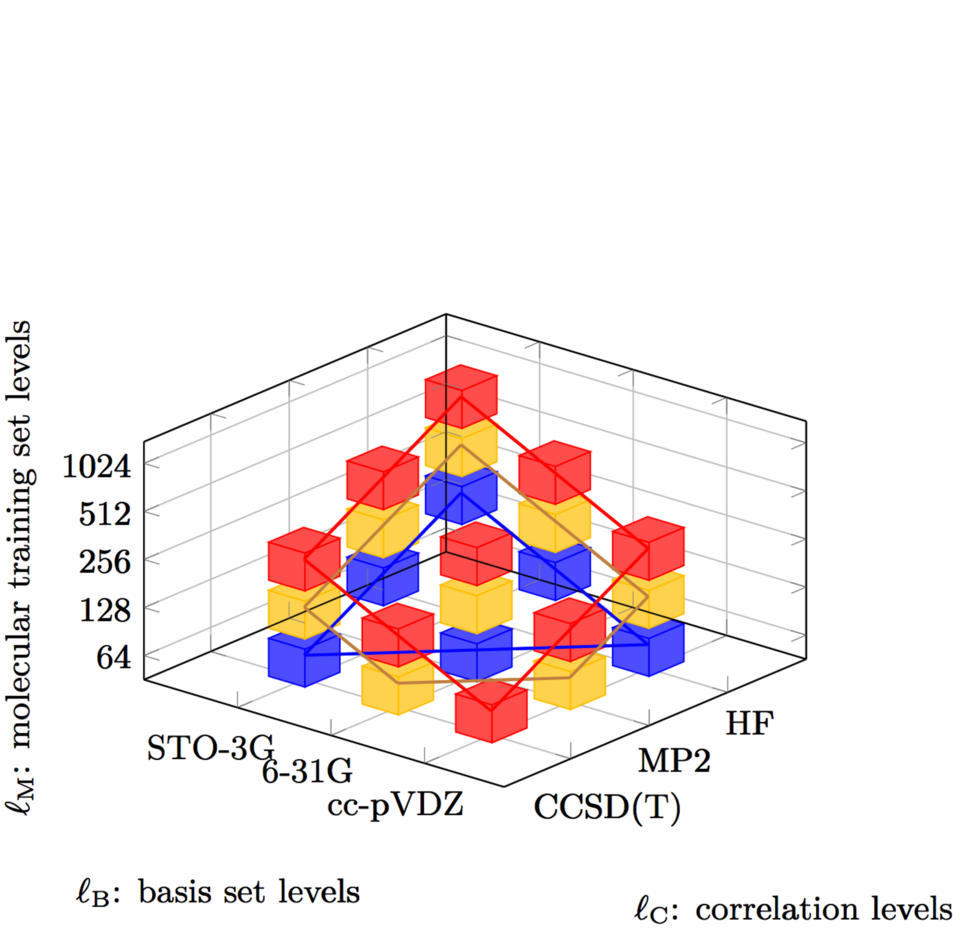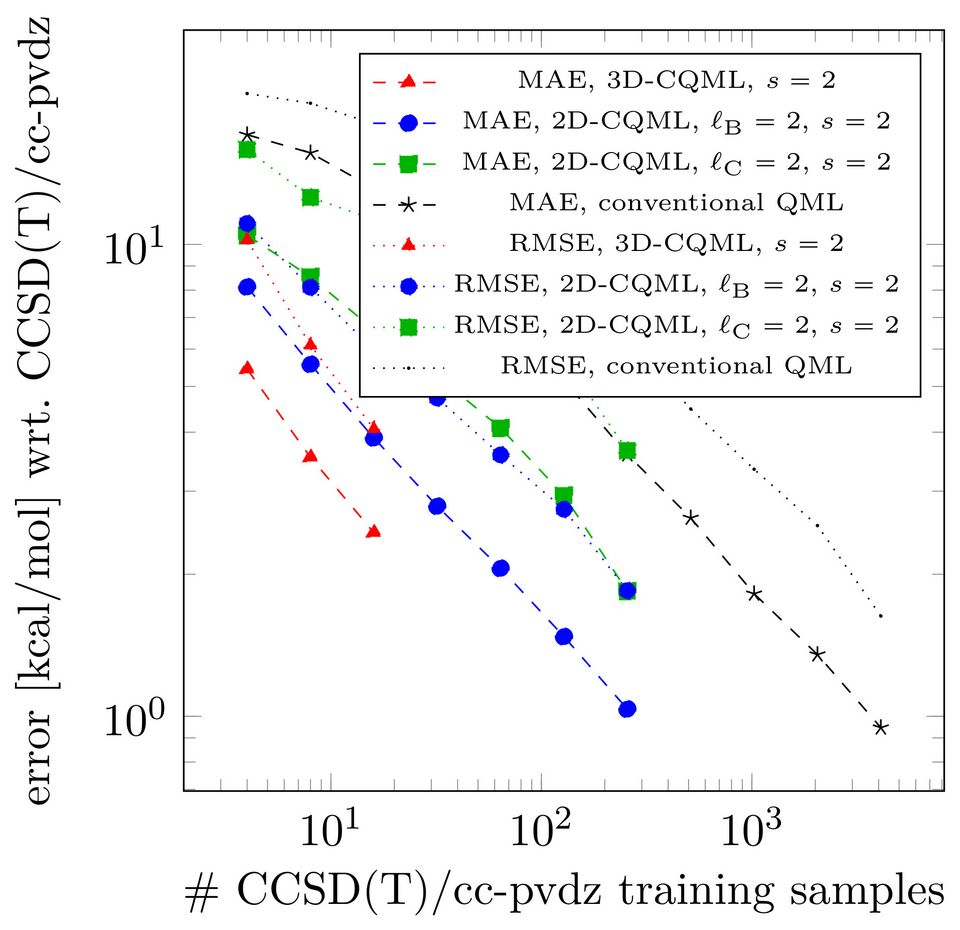## Statement

In the machine learning task "regression", we would like to "train" a model that explains the mapping of given high-dimensional input space X to observations in an observation space Y. That is, the trained model is supposed to give predictions for unseen input in X such that, for a query input, we get a prediction that follows the hidden relation between X and Y .

My main interest is in "kernel ridge regression", which is equivalent to approximation in reproducing kernel Hilbert spaces with regularization. My specific interest is in multi-fidelity learning, fast training methods and applications in scientific computing.

## Exemplary results

#### Multi-fidelity machine learning in quantum chemistry# Subspaces involved in the sparse grid combination technique for kernel ridge regression